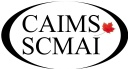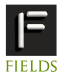June 24-28, 2012 The 2012 Annual Meeting of the Canadian Applied and Industrial Mathematics SocietyToronto Information Contact Us programs(PUT_AT_SIGN_HERE)fields.utoronto.caMini Symposium Applications of Computer Algebra in Applied and Industrial Mathematics

by Marc Moreno Maza, University of Western Ontario
Coauthors: Changbo Chen, University of Western Ontario and Rong Xiao, University of Western Ontario

Computer Algebra Systems (CAS), such as Axiom, Maple, Mathemagix, Mathematica, are powerful software tools which support the work of mathematicians and engineers in many disciplines. Recent algorithmic developments in the context of hardware acceleration technologies are boosting the performances of CAS. This mini-symposium aims at exploring the application of computer algebra to applied and industrial mathematics.

We are particularly interested into works, such as application-driven software packages that provide an interface between computer algebra and other domains. Examples of application areas are: analysis of dynamical systems, cryptography, discrete and continuous optimization, modeling and simulation, to name a few.

PART 1 (Tuesday 26 June PM) "Discrete maths and applications"

1. Rong Xiao, University of Western Ontario
Generating Program Invariants via Polynomial Interpolation

This article focuses on automatically generating polynomial equations that are inductive loop invariants of computer programs.
We propose a new algorithm for this task, which is based on polynomial interpolation. Though the proposed algorithm is not complete, it is efficient and can be applied to a broader range
of problems compared to existing methods targeting similar problems. The efficiency of our approach is testified by experiments on a large collection of programs.
The current implementation of our method is based on polynomial interpolation, for which a total degree bound is needed. Ou the theoretical front, we study the degree and dimension of the invariant ideal of loops which have no branches and where the assignments define a P-solvable recurrence. In addition, we obtain sufficient conditions for non-trivial polynomial equation invariants to exist (resp. not to exist).
(This is a joint work with Marc Moreno Maza.)

2.Gordon Uszkay presenting for
Jacques Carette, McMaster
From Computer Algebra to Property-based Testing Strategies forFunctional Programs.

Starting from the theory of Species, which underlies much of Maple's construct package, we find applications which seems far afield: mostly automated testing of properties of functional programs. Using the theory as a strong guide, we have developed a set of compositional strategies for structured data generation, with the principal use being to test that polymorphic functional programs obey their specification.
This is joint work with Gordon Uszkay.

3. Eric Schost,University of Western Ontario
Lifting techniques for list decoding

Following Sudan's seminal contribution, list decoding algorithms rely on a combination of interpolation and root finding techniques. Augot and Pecquet already showed that Newton iteration is well suited to handle the root-finding
step. We present a lifting operator that generalizes this idea to the recently introduced case of folded codes.
This is a joint work with M. F. I. Chowdhury

4. Esmaeil Mehrabi, University of Western Ontario
Lifting techniques for resultant computation

Computing resultants is a fundamental algorithmic question, at the heart of higher-level algorithms for solving systems of equations, computational topology, etc. However, in many situations, the best known algorithms are still sub-optimal. In this talk, we will show how to improve existing algorithms in a few important cases (e.g., for
polynomials in Z[x,y]), under a few genericity assumptions
(joint work with Romain Lebreton and Eric Schost)

PART 2 (Wednesday 27 June AM)
"System solving and applications"

5.Changbo Chen, University of Western Ontario
Quantifier elimination via triangular decomposition

At ISSAC 2009, the authors presented an algorithm for computing cylindrical algebraic decomposition based on triangular decompositions of polynomial systems. The main difference with respect to Collins' original algorithm is that the concept of cylindrical decomposition of the complex space is introduced, which creates a new framework for computing CADs. In this paper, we investigate how this new framework can be used to do quantifier elimination. We propose an operation that refines a given cylindrical decomposition with a new constraint, equation or inequation. This yields an incremental algorithm for computing the cylindrical decomposition of the complex space. This refinement operation also leads us to a general quantifier elimination procedure based on triangular decompositions. Finally, we obtain a systematic solution for the problem of making use of equational constraints for doing QEs.

6. Andrew Delong, The University of Western Ontario
Automatic Quantifier Elimination Proves a Key Result in Submodular Function Minimization

We show how symbolic computation can automatically prove an important and well-known result in combinatorial optimization. Specifically, we address the question "Which objective functions can be minimized by reduction to s-t min cut?" This question has been answered by a number of highly-cited papers in discrete mathematics and computer vision. By posing this same question to a computer algebra system, we automatically identify submodularity as being the key condition for reduction to s-t min cut.

7. David Wilson, University of Bath
Choice of formulation in Cylindrical Algebraic Decomposition problems

Abstract: Cylindrical Algebraic Decompositions can be used to solve many problems, such as quantifier elimination. For a given QE problem there may be alternative formulations of the Tarski formula that lead to (structurally different) CADs that can still be used to solve the original problem. In this manner the user can utilize the structure of the problem even when using 'abstract' CAD algorithms (that take a list of polynomials as input). Ideally a new formulation should take minimal time to reformulate but offer a large drop in the complexity of the CAD. Some methods of reformulating problems will be discussed as well as potential metrics that may help to decide whether reformulation will be beneficial.
This talk is based on joint work conducted at the University of Bath with Professor James Davenport and Dr. Russell Bradford.

8. Thomas Wolf, Brock University
Recent extensions of the package CRACK for the solution of
non-polynomial differential systems of equations

Applications in Applied and Industrial Mathematics are often
not "clean". For example, they may require the solution of equations for unknowns that depend on a different number of independent variables, that involve algebraic numbers and transcendental functions. A recent series of applications required the solution of systems of differential equations with parametric exponents that had to be computed too. Such applications included traveling waves, conservation laws for complex MKdV-type equations and the symmetry analysis and exact solutions of semilinear heat flow in multi-dimensions. After giving an overview of the computer algebra package CRACK and an introduction to these applications the talk describes extensions that support the solution of differential systems with unknown constants in exponents.

PART 3 (Wednesday 27 June PM) "Modeling and Simulation"

9. Marc Moreno Maza, University of Western Ontario
Exact Computation of the Real Solutions of Polynomial Systems: Software Tools and Applications

Providing an exact and complete description of the real solutions of a given polynomial systems is a challenging question in many respects (theory, algorithms, implementation). Available solutions used to be either of limited practical efficiency or not even suitable for software implementation. Recent advances in algorithms for symbolic computation make it possible today to solve systems of polynomial equations, inequations and inequalities that are of interest in mathematical sciences and engineering. The RegularChains library in the computer algebra system Maple implement these algorithms that we illustrate through a series of applications taken from the study of dynamical systems, non-linear optimization and modeling.
This is a joint work with Changbo Chen, James H. Davenport, Rong Xiao and Bican Xia.

10., 11. Laurent Bernardin, Maplesoft
Computer Algebra for Modeling and Simulation

Modeling and Simulation of Physical Systems is a crucial topic in engineering, driving innovation in a highly competitive environment with increasingly complex product requirements. Traditionally, modeling and simulation problems are treated using numeric methods. It turns out that introducing computer algebra methods both expands the range of models that can be described and leads to significant speed gains in the numeric simulation phase. Using MapleSim as the example, we'll look at how combining numeric and symbolic techniques allows us to handle previously intractable models. In doing so, we will look at a number of examples from industry where these techniques have successfully pushed the boundaries of engineering.

12. David Jeffrey, University of Western Ontario